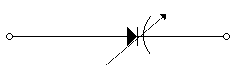Courses

# Test: Electronic Devices And Circuits - 8

## 25 Questions MCQ Test Electronic Devices | Test: Electronic Devices And Circuits - 8

Description
This mock test of Test: Electronic Devices And Circuits - 8 for Electrical Engineering (EE) helps you for every Electrical Engineering (EE) entrance exam. This contains 25 Multiple Choice Questions for Electrical Engineering (EE) Test: Electronic Devices And Circuits - 8 (mcq) to study with solutions a complete question bank. The solved questions answers in this Test: Electronic Devices And Circuits - 8 quiz give you a good mix of easy questions and tough questions. Electrical Engineering (EE) students definitely take this Test: Electronic Devices And Circuits - 8 exercise for a better result in the exam. You can find other Test: Electronic Devices And Circuits - 8 extra questions, long questions & short questions for Electrical Engineering (EE) on EduRev as well by searching above.
QUESTION: 1

Solution:
QUESTION: 2

Solution:
QUESTION: 3

### When a p-n junction is forward biased. The width of depletion layer decreases.

Solution:
QUESTION: 4

In which of the following case the rating of the transformer to deliver 100 watts of d.c. power to a load, will be least?

Solution:
QUESTION: 5

When a p-n Junction is forward biased

Solution:
QUESTION: 6

In a JFET, the drain current is maximum when

Solution:
QUESTION: 7

A potential difference is developed across a current carrying metal strip when the strip is placed in a transverse magnetic field. The above effect is known as

Solution:
QUESTION: 8

In a zener diode

Solution:
QUESTION: 9

Which one of the following bipolar transistors has the highest current gain bandwidth Product (fr) for similar geometry?

Solution:
QUESTION: 10

The depletion layer across a P+ n junction lies

Solution:
QUESTION: 11

An increase of reverse voltage decreases the junction capacitance.

Solution:
QUESTION: 12

The maximum forward current in case of signal diode is in the range of

Solution:
QUESTION: 13

On which of the following effect do thermocouples work?

Solution:
QUESTION: 14

Which of the following constitutes an active component?

Solution:
QUESTION: 15

Which of the following characteristics of a silicon p-n junction diode make it suitable for use as ideal diode?

1. It has low saturation current.
2. It has high value of cut in voltage.
3. It can withstand large reverse voltage.
4. When compared with germanium diode, silicon diode shows a lower degree of temperature dependence under reverse conditions.
Select the answer using the given below
Solution:
QUESTION: 16

Resistivity of carbon is around

Solution:
QUESTION: 17

Which of the following is anti-ferromagnetic material?

Solution:
QUESTION: 18

Assertion (A): The capacitance of a reverse biased pin diode is lower than that of reverse biased p-n diode.

Reason (R): A PIN diode has an intrinsic layer between p and n regions.

Solution:
QUESTION: 19

In the saturation region of CE output characteristics of n-p-n transistor, VCE is about

Solution:
QUESTION: 20

In n channel JFET

Solution:
QUESTION: 21

Figure represents aSolution:
QUESTION: 22

Almost all resistors are made in a monolithic integrated circuit

Solution:
QUESTION: 23

The forbidden energy gap for silicon is

Solution:
QUESTION: 24

In energy band diagram of n type semiconductor, the donor energy level is

Solution:
QUESTION: 25

For an n-channel JEFT having drain source voltage constant if the gate source voltage is increased (more negative) pinch off would occur for

Solution: